Download Presentation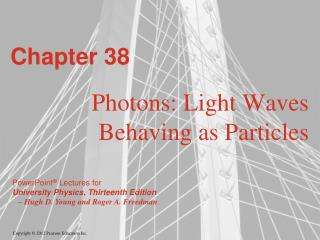Chapter 38

# Chapter 38 - PowerPoint PPT Presentation

Chapter 38. Photons: Light Waves Behaving as Particles. Goals for Chapter 38. To consider the fundamental constituent of light, the photon To study the removal of an electron by an incident photon, the photoelectric effectI am the owner, or an agent authorized to act on behalf of the owner, of the copyrighted work described.
Download Presentation## Chapter 38

An Image/Link below is provided (as is) to download presentation

Download Policy: Content on the Website is provided to you AS IS for your information and personal use and may not be sold / licensed / shared on other websites without getting consent from its author.While downloading, if for some reason you are not able to download a presentation, the publisher may have deleted the file from their server.

- - - - - - - - - - - - - - - - - - - - - - - - - - E N D - - - - - - - - - - - - - - - - - - - - - - - - - -
Presentation Transcript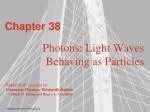### Chapter 38

Photons: Light Waves Behaving as Particles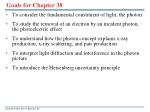Goals for Chapter 38
• To consider the fundamental constituent of light, the photon
• To study the removal of an electron by an incident photon, the photoelectric effect
• To understand how the photon concept explains x-ray production, x-ray scattering, and pair production
• To interpret light diffraction and interference in the photon picture
• To introduce the Heisenberg uncertainty principle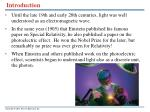Introduction
• Until the late 19th and early 20th centuries, light was well understood as an electromagnetic wave.
• In the same year (1905) that Einstein published his famous paper on Special Relativity, he also published a paper on the photoelectric effect. He won the Nobel Prize for the later, but remarkably no prize was ever given for Relativity!
• When Einstein and others published work on the photoelectric effect, scientists began to understand light also as a discrete unit, the photon.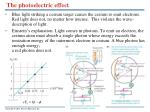The photoelectric effect
• Blue light striking a cesium target causes the cesium to emit electrons. Red light does not, no matter how intense. This violates the wave-description of light.
• Einstein’s explanation: Light comes in photons. To emit an electron, the cesium atom must absorb a single photon whose energy exceeds the ionization energy of the outermost electron in cesium. A blue photon has enough energy; a red photon does not.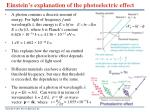Einstein’s explanation of the photoelectric effect
• A photon contains a discrete amount of energy. For light of frequency f and wavelength , this energy is E = hf orE = (hc)/l, where h is Planck’s constant 6.626 × 10−34 J-s = 4.136 × 10−15 eV-s.
• 1 eV = 1.602 × 10−19 J-s
• This explains how the energy of an emitted electron in the photoelectric effect depends on the frequency of light used.
• Different materials can have a different threshold frequency, but once that threshold is exceeded, the dependence is the same.
• The momentum of a photon of wavelength  is p = E/c = h/. Can write it in terms of wavenumber k = 2p/l, or wave-vector , where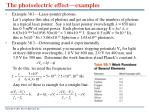The photoelectric effect—examples
• Example 38.1—Laser-pointer photons.
• Let’s explore this idea of photons and get an idea of the numbers of photons in a typical laser pointer. Say a red laser pointer (wavelength l = 650 nm) has 0.5 mW of output power. Each photon has an energy E = hc/ l, = 6.63×10-34*3×108/6.50×10-7 = 3.06×10-19 J. Since 0.5 mW = 5×10-3 J/s, this is 5×10-3 J/s / 3.06×10-19 J/photon = 1.63×1016 photons/s.
• Example 38.3—Determining  and h experimentally.
• In a photoelectric experiment you measure stopping potentials V0 for light of three different wavelengths, 1.0 V for 600 nm, 2.0 V for 400 nm, and 3.0 V for 300 nm. Determine the work function f and Planck’s constant h.
• To do this, we need the frequencies
• Any two of these is enough for a straight line.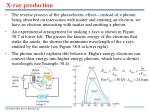X-ray production
• The reverse process of the photoelectric effect—instead of a photon being absorbed on interaction with matter and emitting an electron, we have an electron interacting with matter and emitting a photon.
• An experimental arrangement for making x rays is shown in Figure 38.7 at lower left. The greater the kinetic energy of the electrons that strike the anode, the shorter the minimum wavelength of the x rays emitted by the anode (see Figure 38.8 at lower right).
• The photon model explains this behavior: Higher-energy electrons can convert their energy into higher-energy photons, which have a shorter wavelength (see Example 38.4).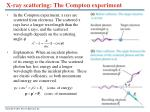X-ray scattering: The Compton experiment
• In the Compton experiment, x rays are scattered from electrons. The scattered x rays have a longer wavelength than the incident x rays, and the scattered wavelength depends on the scattering angle .
• Explanation: When an incident photon collides with an electron, it transfers some of its energy to the electron. The scattered photon has less energy and a longer wavelength than the incident photon. These are elastic collisions, so both momentum and energy are conserved.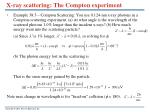X-ray scattering: The Compton experiment
• Example 38.5—Compton Scattering: You use 0.124-nm x-ray photons in a Compton-scattering experiment. (a) At what angle is the wavelength of the scattered photons 1.0% longer than the incident x-rays? (b) How much energy went into the scattering particle?

(a) Since l′ is 1% longer, l′ – l = 0.01l, so solving for f,

(b) The photon energy lost in the scattering is

That is the same energy gained by the particle.

Note max change in wavelength is for “head-on” collision (f= 180),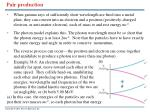Pair production
• When gamma rays of sufficiently short wavelength are fired into a metal plate, they can convert into an electron and a positron (positively-charged electron, or anti-matter electron), each of mass m and rest energy mc2.
• The photon model explains this: The photon wavelength must be so short that the photon energy is at least 2mc2. Note that the particles have to have exactly the same energy and angle in order to conserve momentum.
• The reverse process can occur—the positron and electron can come together and annihilate to produce a photon (or more than one photon)
• Example 38.6: An electron and positron,

initially far apart, move toward each other at the

same speed. They collide head-on, annihilating

each other and producing two photons. Find the

energies, wavelengths and frequencies of the two

photons if the initial kinetic energies of the two are

(a) negligible, or (b) both 5.00 MeV.

(a) In this case, the energies of the particles are each

just their rest masses mc2, so each photon has that energy.Diffraction and uncertainty
• When a photon passes through a narrow slit, its momentum becomes uncertain and the photon can deflect to either side. By limiting the size vertical space to the slit width a, the vertical (y) momentum becomes uncertain according to the Heisenberg Uncertainty Principle:
• Each photon lands with some uncertainty, but a diffraction pattern is the result of many photons hitting the screen. The pattern appears even if only one photon is present at a time in the experiment.Tthe pattern is not a consequence of interference between two photons. Each photon interferes with itself.The Heisenberg Uncertainty Principle
• You cannot simultaneously know the position and momentum of a photon with arbitrarily great precision. The better you know the value of one quantity, the less well you know the value of the other.
• In addition, the better you know the energy of a photon, the less well you know when you will observe it.

No localization

in space

Better localization

in space

Precise knowledge

of wavelength

(momentum)

Worse knowledge

of wavelength

(momentum)